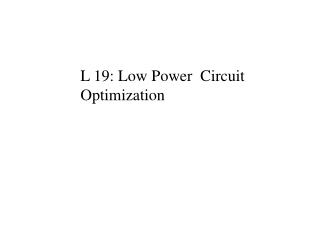DownloadDownload PresentationL 19: Low Power Circuit Optimization

# L 19: Low Power Circuit Optimization

Download Presentation## L 19: Low Power Circuit Optimization

- - - - - - - - - - - - - - - - - - - - - - - - - - - E N D - - - - - - - - - - - - - - - - - - - - - - - - - - -
##### Presentation Transcript

1. L 19: Low Power Circuit Optimization

2. Power Optimization • Modeling and Technology • Circuit Design Level • logic Families • low-power Flip-Flops • low-power clock distribution • Logic and Module Design Level • Architecture and System Design Level • Some Design Examples

3. Choice of Logic Family • Power delay product improves as supply voltage decreases • The best logic style minimizes power-delay product

4. Static CMOS Full Adder VDD C’ C B A B B’ B’ B A C A A’ A C carry A’ A’ sum B A B B’ B B C C’

5. NO Race dynamic CMOS logic(NORA) • Full Adder phi phi’ A C A A B C B A B B C carry phi sum phi’ Phi’ phi

6. Cascode Voltage Switch Logic(CVSL) Full Adder phi phi phi phi sum’ sum A A’ A Carry’ Carry A A’ B B’ B B B’ B B’ C C’ C C’ phi phi

7. Differential Cascode Voltage Switch Logic • (DCVSL) sum’ sum Carry’ Carry A A’ A A’ A B B’ B B’ B B’ B C C’ C C’

8. CMOS NonThreshold Logic(CNTL) Full Adder Carry’ Carry sum’ sum A A’ A A’ A B B’ B B’ B B’ B C C’ C C’

9. Logic Family Transistors Rank Area(m2) Rank • Full Adder Transistor Count and Area CMOS 30 4 21,294 3 NORA 22 1 14,319 1 CVSL 24 3 25,740 4 DCVS 22 1 21,080 2 CNTL 34 5 40,020 5 • Delays when 16-bit ripple carry adder was made Simulated(ns) Rank Measured(ns) Rank CMOS 46.34 3 60 3 NORA 45.9 2 47.2 1 CVSL 45.4 1 49.2 2 DCVS 61.5 5 72.6 4 CNTL 54.1 4 87.0 5

10. Logic Family • Peak switching current simulated(mA) Rank Measure(mA) Rank CMOS 2.42 4 1.30 5 NORA 2.74 5 1.20 3 CVSL 1.08 1 1.06 1 DCVS 1.19 2 1.22 4 CNTL 1.25 3 1.18 2 • Average measures current Current(A) Rank CMOS 98 1 NORA 948 4 CVSL 925 3 DCVS 116 2 CNTL 1320 5

11. A Complementary Pass Transistor Logic(CPL) A A’ Z Z’ B B’ A’ A B A’ B’ Z (AB)’ AB Z’ Q’ Q

12. A High Level Degradation B’ A’ VDD VDD VDD B B’ VDD-VTN AB (AB)’

13. A B’ B A’ AB Dual Path Transistor Logic(DPL) A’ B B’ A (AB)’

14. A Swing Restored Pass Transistor • Logic(SRPL) B B’ A’ NMOS CPL Network B B’ O O’ AB (AB)’

15. Low-Power Flip-Flops • Power consumption of Flip-Flop • power consumed for the internal state change • power consumed for the clocking • Because the stage change is infrequent, the clocking capacitance must be reduced. • Conventional C2MOS Flip-Flop At each clock, the switched capacitance is 10MOS gates

16. Low-Power Flip-Flops D D Q • Gated D Flip-Flop • SSTC(Static Single-Transistor Clocked Flip-Flop At each clock, the switched capacitance 2 MOS gates, but Slow and about 40 transistors Clk CK The switched capacitance is 2 MOS gates and 16 transistors

17. Power Optimization • Modeling and Technology • Circuit Design Level • Logic and Module Design Level • logic synthesis • module design optimization • Architecture and System Design Level • Some Design Examples

18. Power dissipation layout 2um CMOS MOSIS condition 1,000 pseudo-random input averaging the result Result CSA has lowest power dissipation power supply current falls to zero faster Adder Type Delay (nsec) Area (mm2) Current (mW) Ripple Carry 54.27 0.2527 0.117 Carry Skip-I 28.38 0.4492 0.109 Carry Skip-II 21.84 0.5149 0.126 Carry Lookahead 17.13 0.7454 0.171 Carry Select 19.56 1.0532 0.216 Conditional Sum 20.05 1.4784 0.304 Module Design Optimization • Arithmetic component : Adder • Carry Skip-I : constant block size • Carry Skip-II : variable block size

19. Power estimation conditions 50,000 random distribution input Result wallace multiplier more attractive when operand size is large irregular layout and large layout area array multiplier becomes unattractive as operand size increase has higher average number of logic transitions has much higher delay Multiplier Type Delay(in gate) Size(gates) Transition Modified Array 8b 50 567 583 16b 98 2,405 7,348 32b 198 9,918 99,102 Wallace 8b 35 613 573 16b 51 2,569 3,874 32b 63 10,413 19,548 Module Design Optimization • Arithmetic component : Multiplier